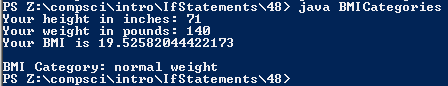# Assignment #48 and BMI Categories

## Code

```    /// Name: Brendan Baird
/// Period: 6
/// Program Name: BMI Categories
/// File Name: BMICategories.java
/// Date Finished: 10/22/2015

import java.util.Scanner;

public class BMICategories
{

public static void main(String[] args)
{

Scanner keyboard = new Scanner(System.in);
double m, kg, inches, pounds, bmi;
String category = "";

inches = keyboard.nextDouble();

m = inches * 0.0254;

pounds = keyboard.nextDouble();

kg = pounds * 0.453592;

bmi = kg / (m*m);

System.out.println("Your BMI is " + bmi);

if (bmi < 15.0)
category = "very severely underweight";
if (bmi >= 15.0 && bmi <= 16.0)
category = "severely underweight";
if (bmi >= 16.1 && bmi <= 18.4)
category = "underweight";
if (bmi >= 18.5 && bmi <= 24.9)
category = "normal weight";
if (bmi >= 25.0 && bmi <= 29.9)
category = "overweight";
if (bmi >= 30.0 && bmi <= 34.9)
category = "moderately obese";
if (bmi >= 35.0 && bmi <= 39.9)
category = "severely obese";
if (bmi >= 40.0)
category = "very severely obese";

System.out.println();
System.out.println("BMI Category: " + category);
}
}
```

### Picture of the output